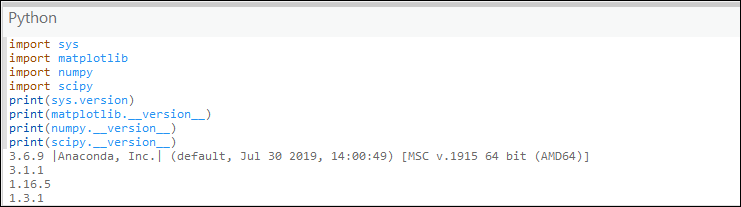English

# FAQ: What version of Python is used in ArcGIS?

## Question

What version of Python is used in ArcGIS?

The Python programming language has been used in each version since ArcGIS 9.0. It is incorporated into the setups of ArcGIS Desktop, ArcGIS Pro, and ArcGIS Enterprise. ArcGIS installs the versions of Python listed below.

Also included for most recent releases is the version of NumPy and matplotlib included with the Python environment.

```Note:
It is not recommended to install an independent version of Python for use in ArcGIS Desktop. Using a different version of Python can lead to compatibility issues and root folder problems. It is not supported to replace the version of Python that ships with ArcGIS Server.```

ArcGIS Pro

The version of Python and its stack versions can be determined by opening the Python window in the application (View > Python) and typing the following commands:

```import sys
import matplotlib
import numpy
import scipy
print(sys.version)
print(matplotlib.__version__)
print(numpy.__version__)
print(scipy.__version__)```

The commands display the default Python version used with the application. In the image below (ArcGIS Pro 2.5), the version of Python is 3.6.9, followed by the versions of the matplotlib, numpy, and scipy packages. Many other packages are also included with the Python environment. You can check the version of those packages using the Python tab in the ArcGIS Pro settings.```Note:
In ArcGIS Pro versions 1.0 through 1.2, if Python was used outside of ArcGIS Pro, it was required to install Python for ArcGIS Pro. This is not required for versions 1.3 and later.```

ArcGIS Desktop

• 10.8.1 - Python 2.7.18 and NumPy 1.9.3
• 10.8 - Python 2.7.16 and NumPy 1.9.3
• 10.7.1 - Python 2.7.16 and NumPy 1.9.3
• 10.7 - Python 2.7.15 and NumPy 1.9.3
• 10.6.1 - Python 2.7.14 and NumPy 1.9.3
• 10.6 - Python 2.7.14 and NumPy 1.9.3
• 10.5.1 - Python 2.7.13 and NumPy 1.9.3
• 10.5 - Python 2.7.12 and NumPy 1.9.3
• 10.4.x - Python 2.7.10 and NumPy 1.9.2
• 10.3.x - Python 2.7.8 and NumPy 1.7.1
• 10.2.1 and 10.2.2 - Python 2.7.5 and NumPy 1.7.1
• 10.2 - Python 2.7.3 and NumPy 1.6.1
• 10.1 - Python 2.7.2 and NumPy 1.6.1
• 10.0 - Python 2.6.5 and NumPy 1.3.0

ArcGIS Enterprise

ArcGIS Enterprise also ships with Python. In recent releases, both Python 2.x and Python 3.x runtimes are provided.

ArcGIS Enterprise

• 10.8.1
• Python 2.7.18, NumPy 1.9.3, and mayplotlib 1.5.2
• Python 3.6.10, NumPy 1.18.1, and matplotlib 3.2.1
• 10.8
• Python 2.7.16, NumPy 1.9.3, and matplotlib 1.5.2
• Python 3.6.9, NumPy 1.16.5, and matplotlib 3.1.1
• 10.7.1
• Python 2.7.16, NumPy 1.9.3, and matplotlib 1.5.2
• Python 3.6.8, NumPy 1.16.2, matplotlib 3.0.3
• 10.7
• Python 2.7.15, NumPy 1.9.3, and matplotlib 1.5.2
• Python 3.6.6, NumPy 1.15.1, matplotlib 2.2.3
• 10.6.1
• Python 2.7.14, NumPy 1.9.3, and matplotlib 1.5.2
• Python 3.6.5, NumPy 1.14.2, matplotlib 2.2.2
• 10.6
• Python 2.7.14, NumPy 1.9.3, and matplotlib 1.5.2
• Python 3.6.2
• 10.5.1
• Python 2.7.13, NumPy 1.9.3, and matplotlib 1.5.2
• Python 3.5.3
• 10.5
• Python 2.7.12, NumPy 1.9.3, and matplotlib 1.5.2
• Python 3.5.2
• 10.4 and 10.4.1
• Python 2.7.10, NumPy 1.9.2, and matplotlib 1.4.3
• Python 3.4.1
• 10.3.x - Python 2.7.8, NumPy 1.7.1, and matplotlib 1.3.0
• 10.2.1 and 10.2.2 - Python 2.7.5 and NumPy 1.7.1
• 10.2 - Python 2.7.3 and NumPy 1.6.1
• 10.1 - Python 2.7.2 and NumPy 1.6.1
• 10.0 - Python 2.6.5 and NumPy 1.3.0

ArcGIS Notebook Server
Each notebook runtime in ArcGIS Notebook Server packages a precise list of Python libraries, including a specific version of each. If you need a library that is not in either runtime by default, you can extend a notebook runtime to include it. See the ArcGIS Notebook Server Online Help Available Python Libraries for a complete list of the Python libraries packaged in each default runtime

## Related Information

Last Published: 6/26/2020

Article ID: 000013224

Software: ArcGIS Pro 2.5.2, 2.5.1, 2.5, 2.4.3, 2.4.2, 2.4.1, 2.4, 2.3.3, 2.3.2, 2.3.1, 2.3, 2.2.4, 2.2.3, 2.2.2, 2.2.1, 2.2, 2.1.3, 2.1.2, 2.1.1, 2.1, 2.0.1, 2.0, 1.4.1, 1.4, 1.3.1, 1.3, 1.2 ArcMap 10.8, 10.7.1, 10.7, 10.6.1, 10.6, 10.5.1, 10.5, 10.4.1, 10.4, 10.3.1, 10.3, 10.2.2, 10.2.1, 10.2, 10.1, 10